I’ll show you how to calculate the ‘order flow imbalance’ and build a high-frequency trading signal with the results. We’ll see if it is a profitable strategy and how it might be useful as a market indicator.

Enjoy these types of posts? Then you should sign up for my newsletter. It’s a short monthly recap of anything and everything I’ve found interesting recently plus any posts I’ve written. So sign up and stay informed!

A couple of months ago I attended the Oxford Math Finance seminar where there was a presentation on order book dynamics to predict short-term price direction. Nicholas Westray presented Deep Order Flow Imbalance: Extracting Alpha at Multiple Horizons from the Limit Order Book. By using deep learning they predict future price movements using common neural network architectures such as the basic multi-layer perceptron (MLP), Long Term Short Memory network (LSTM) and convolutional neural networks (CNN). Combining all three networks types lets you extract the strengths of each network:

• CNNs: Reduce frequency variations.
• The variations between each input reduce to some common factors.
• LSTMs: Learn temporal structures
• How are the different inputs correlated with each other such as autocorrelation structure.
• MPLs: Universal approximators.
• An MLP can approximate any function.

A good reference for LSTM-CNN combinations is DeepLOB: Deep Convolution Neural Networks for Limit Order Books where they use this type of neural network to predict whether the market is moving up or down.

The Deep Order Flow talk was an excellent overview of deep learning concepts and described how there is a great overlap between computer vision and the state of the order book. You can build an “image” out of the different order book levels and pass this through the neural networks. My main takeaway from the talk was the concept of Order Flow Imbalance. This is a transformation that uses the order book to build a feature to predict future returns.

I’ll show you how to calculate the order flow imbalance and see how well it predicts future returns.

## The Setup

I have a QuestDB database with the best bid and offer price and size at those levels for BTCUSD from Coinbase over roughly 24 hours. To read how I collected this data check out my previous post on streaming data into QuestDB.

Julia easily connects to QuestDB using the LibPQ.jl package. I also load in the basic data manipulation packages and some statistics modules to calculate the necessary values.

using LibPQ
using DataFrames, DataFramesMeta
using Plots
using Statistics, StatsBase
using CategoricalArrays
using Dates
using RollingFunctions

conn = LibPQ.Connection("""
dbname=qdb
host=127.0.0.1
port=8812


Order flow imbalance is about the changing state of the order book. I need to pull out the full best bid best offer table. Each row in this table represents when the best price or size at the best price changed.

bbo = execute(conn,
"SELECT *
FROM coinbase_bbo") |> DataFrame
dropmissing!(bbo);


I add the mid-price in too, as we will need it later.

bbo = @transform(bbo, mid = (:ask .+ :bid) / 2);


It is a big dataframe, but thankfully I’ve got enough RAM.

## Calculating Order Flow Imbalance

Order flow imbalance represents the changes in supply and demand. With each row one of the price or size at the best bid or ask changes which corresponds to change in the supply or demand, even at a high frequency level, of Bitcoin.

• Best bid or size at the best bid increase -> increase in demand.
• Best bid or size at the best bid decreases -> decrease in demand.
• Best ask decreases or size at the best ask increases -> increase in supply.
• Best ask increases or size at the best ask decreases -> decrease in supply.

Mathematically we summarise these four effects at from time $$n-1$$ to $$n$$ as:

$e_n = I_{\{ P_n^B \geq P^B_{n-1} \}} q_n^B - I_\{ P_n^B \leq P_{n-1}^B \} q_{n-1}^B - I_\{ P_n^A \leq P_{n-1}^A \} q_n^A + I_\{ P_n^A \geq P_{n-1}^A \} q_{n-1}^A,$

where $$P$$ is the best price at the bid ($$P^B$$) or ask ($$P^A$$) and $$q$$ is the size at those prices.

Which might be a bit easier to read as Julia code:

e = Array{Float64}(undef, nrow(bbo))
fill!(e, 0)

for n in 2:nrow(bbo)

e[n] = (bbo.bid[n] >= bbo.bid[n-1]) * bbo.bidsize[n] -
(bbo.bid[n] <= bbo.bid[n-1]) * bbo.bidsize[n-1] -

end

bbo[!, :e] = e;


To produce an Order Flow Imbalance (OFI) value, you need to aggregate $$e$$ over some time-bucket. As this is a high-frequency problem I’m choosing 1 second. We also add in the open and close price of the buckets and the return across this bucket.

bbo = @transform(bbo, timestampfloor = floor.(:timestamp, Second(1)))
bbo_g = groupby(bbo, :timestampfloor)
modeldata = @combine(bbo_g, ofi = sum(:e), OpenPrice = first(:mid), ClosePrice = last(:mid), NTicks = length(:e))
modeldata = @transform(modeldata, OpenCloseReturn = 1e4*(log.(:ClosePrice) .- log.(:OpenPrice)))
modeldata = modeldata[2:(end-1), :]
first(modeldata, 5)


5 rows × 6 columns

timestampfloorofiOpenPriceClosePriceNTicksOpenCloseReturn
DateTimeFloat64Float64Float64Int64Float64
12021-07-24T08:50:360.075315933655.133655.1770.0
22021-07-24T08:50:374.44089e-1633655.133655.1470.0
32021-07-24T08:50:380.033655.133655.1200.0
42021-07-24T08:50:393.0572733655.133655.11640.0
52021-07-24T08:50:402.4041733655.133657.42780.674467

Now we do the usual train/test split by selecting the first 70% of the data.

trainInds = collect(1:Int(floor(nrow(modeldata)*0.7)))
trainData = modeldata[trainInds, :]
testData = modeldata[Not(trainInds), :];


We are going to fit a basic linear regression using the OFI value as the single predictor.

using GLM

ofiModel = lm(@formula(OpenCloseReturn ~ ofi), trainData)

StatsModels.TableRegressionModel{LinearModel{GLM.LmResp{Vector{Float64}}, GLM.DensePredChol{Float64, LinearAlgebra.CholeskyPivoted{Float64, Matrix{Float64}}}}, Matrix{Float64}}

OpenCloseReturn ~ 1 + ofi

Coefficients:
─────────────────────────────────────────────────────────────────────────────
Coef.   Std. Error       t  Pr(>|t|)  Lower 95%   Upper 95%
─────────────────────────────────────────────────────────────────────────────
(Intercept)  -0.0181293  0.00231571    -7.83    <1e-14  -0.022668  -0.0135905
ofi           0.15439    0.000695685  221.92    <1e-99   0.153026   0.155753
─────────────────────────────────────────────────────────────────────────────


We see a positive coefficient of 0.15 which is very significant.

r2(ofiModel)

0.3972317963590547


A very high in-sample $$R^2$$.

predsTrain = predict(ofiModel, trainData)
predsTest = predict(ofiModel, testData)

(mean(abs.(trainData.OpenCloseReturn .- predsTrain)),
mean(abs.(testData.OpenCloseReturn .- predsTest)))

(0.3490577385082666, 0.35318460250890665)


Comparable mean absolute error (MAE) across both train and test sets.

sst = sum((testData.OpenCloseReturn .- mean(testData.OpenCloseReturn)) .^2)
ssr = sum((predsTest .- mean(testData.OpenCloseReturn)) .^2)
ssr/sst

0.4104873667550974


An even better $$R^2$$ in the test data

extrema.([predsTest, testData.OpenCloseReturn])

2-element Vector{Tuple{Float64, Float64}}:
(-5.400295917229609, 5.285718311926791)
(-11.602503514716034, 11.46049286770534)


But doesn’t quite predict the largest or smallest values.

So overall:

• Comparable R2 and MAE values across the training and test sets.
• Positive coefficient indicates that values with high positive order flow imbalance will have a large positive return.

But, this all suffers from the cardinal sin of backtesting, we are using information from the future (the sum of the $$e$$ values to form the OFI) to predict the past. By the time we know the OFI value, the close value has already happened! We need to be smarter if we want to make trading decisions based on this variable.

So whilst it doesn’t give us an actionable signal, we know that it can explain price moves, we know just have to reformulate our model and make sure there is no information leakage.

## Building a Predictive Trading Signal

I now want to see if OFI can be used to predict future price returns. First up, what do the OFI values look like and what about if we take a rolling average?

Using the excellent RollingFunctions.jl package we can calculate the five-minute rolling average and compare it to the raw values.

xticks = collect(minimum(trainData.timestampfloor):Hour(4):maximum(trainData.timestampfloor))
xtickslabels = Dates.format.(xticks, dateformat"HH:MM")

ofiPlot = plot(trainData.timestampfloor, trainData.ofi, label = :none, title="OFI", xticks = (xticks, xtickslabels), fmt=:png)
ofi5minPlot = plot(trainData.timestampfloor, runmean(trainData.ofi, 60*5), title="OFI: 5 Minute Average", label=:none, xticks = (xticks, xtickslabels))
plot(ofiPlot, ofi5minPlot, fmt=:png)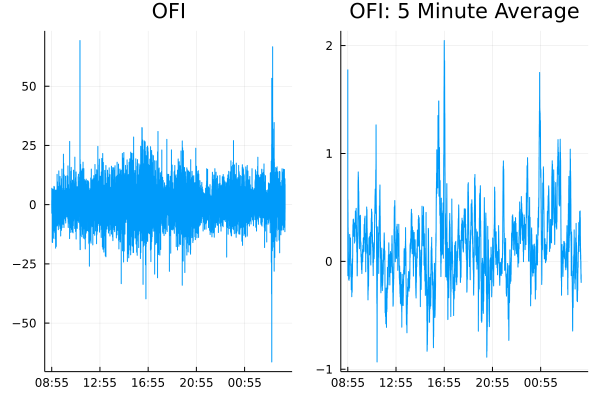It’s very spiky, but taking the rolling average smooths it out. To scale the OFI values to a known range, I’ll perform the Z-score transform using the rolling five-minute window of both the mean and variance. We will also use the close to close returns rather than the open-close returns of the previous model and make sure it is lagged correctly to prevent information leakage.

modeldata = @transform(modeldata, ofi_5min_avg = runmean(:ofi, 60*5),
ofi_5min_var = runvar(:ofi, 60*5),
CloseCloseReturn = 1e4*[diff(log.(:ClosePrice)); NaN])

modeldata = @transform(modeldata, ofi_norm = (:ofi .- :ofi_5min_avg) ./ sqrt.(:ofi_5min_var))

modeldata[!, :CloseCloseReturnLag] = [NaN; modeldata.CloseCloseReturn[1:(end-1)]]

modeldata[1:7, [:ofi, :ofi_5min_avg, :ofi_5min_var, :ofi_norm, :OpenPrice, :ClosePrice, :CloseCloseReturn]]


7 rows × 7 columns

ofiofi_5min_avgofi_5min_varofi_normOpenPriceClosePriceCloseCloseReturn
Float64Float64Float64Float64Float64Float64Float64
10.07531590.07531590.0NaN33655.133655.10.0
24.44089e-160.0376580.00283625-0.70710733655.133655.10.0
30.00.02510530.00189083-0.5773533655.133655.10.0
43.057270.7831462.299771.4995933655.133655.10.674467
52.404171.107352.250370.86447333655.133657.41.97263
62.45361.331722.102360.77373233657.433664.00.252492
7-2.333140.8081733.67071-1.6395933664.033664.9-0.531726
xticks = collect(minimum(modeldata.timestampfloor):Hour(4):maximum(modeldata.timestampfloor))
xtickslabels = Dates.format.(xticks, dateformat"HH:MM")

plot(modeldata.timestampfloor, modeldata.ofi_norm, label = "OFI Normalised", xticks = (xticks, xtickslabels), fmt=:png)
plot!(modeldata.timestampfloor, modeldata.ofi_5min_avg, label="OFI 5 minute Average")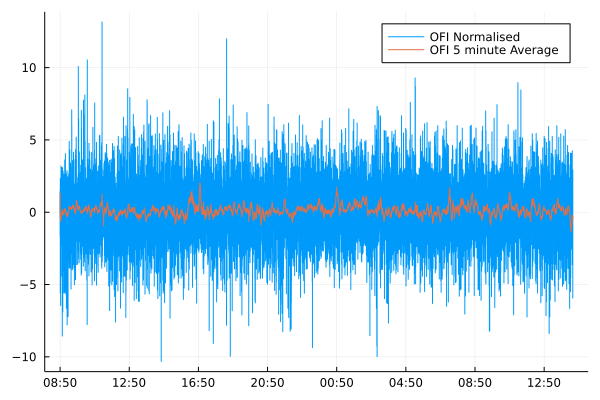The OFI values have been compressed from $$(-50, 50)$$ to $$(-10, 10)$$. From the average values we can see periods of positive and negative regimes.

When building the model we split the data into a training and testing sample, throwing away the early values where the was not enough values for the rolling statistics to calculate.

We use a basic linear regression with just the normalised OFI value.

trainData = modeldata[(60*5):70000, :]
testData = modeldata[70001:(end-1), :]

ofiModel_predict = lm(@formula(CloseCloseReturn ~ ofi_norm), trainData)

StatsModels.TableRegressionModel{LinearModel{GLM.LmResp{Vector{Float64}}, GLM.DensePredChol{Float64, LinearAlgebra.CholeskyPivoted{Float64, Matrix{Float64}}}}, Matrix{Float64}}

CloseCloseReturn ~ 1 + ofi_norm

Coefficients:
────────────────────────────────────────────────────────────────────────────
Coef.  Std. Error      t  Pr(>|t|)    Lower 95%   Upper 95%
────────────────────────────────────────────────────────────────────────────
(Intercept)  0.0020086  0.00297527   0.68    0.4996  -0.00382293  0.00784014
ofi_norm     0.144358   0.00292666  49.33    <1e-99   0.138622    0.150094
────────────────────────────────────────────────────────────────────────────


A similar value in the coefficient compared to our previous model and it remains statistically significant.

r2(ofiModel_predict)

0.033729601695801414


Unsurprisingly, a massive reduction on in-sample $$R^2$$. A value of 3% is not that bad, in the Deep Order Flow paper they achieve values of around 1% but over a much larger dataset and across multiple stocks. My 24 hours of Bitcoin data is much easier to predict.

returnPredictions = predict(ofiModel_predict, testData)

testData[!, :CloseClosePred] = returnPredictions

sst = sum((testData.CloseCloseReturn .- mean(testData.CloseCloseReturn)) .^2)
ssr = sum((testData.CloseClosePred .- mean(testData.CloseCloseReturn)) .^2)
ssr/sst

0.030495583445248473


The out-of-sample $$R^2$$ is also around 3%, so not that bad really in terms of overfitting. It looks like we’ve got a potential model on our hands.

## Does This Signal Make Money?

We can now go through a very basic backtest to see if this signal is profitable to trade. This will all be done in pure Julia, without any other packages.

Firstly, what happens if we go long every time the model predicts a positive return and likewise go short if the model predicts a negative return. This means simply taking the sign of the model prediction and multiplying it by the observed returns will give us the returns of the strategy.

In short, this means if our model were to predict a positive return for the next second, we would immediately buy at the close and be filled at the closing price. We would then close out our position after the second elapsed, again, getting filled at the next close to produce a return.

xticks = collect(minimum(testData.timestampfloor):Hour(4):maximum(testData.timestampfloor))
xtickslabels = Dates.format.(xticks, dateformat"HH:MM")

plot(testData.timestampfloor, cumsum(sign.(testData.CloseClosePred) .* testData.CloseCloseReturn),
label=:none, title = "Cummulative Return", fmt=:png, xticks = (xticks, xtickslabels))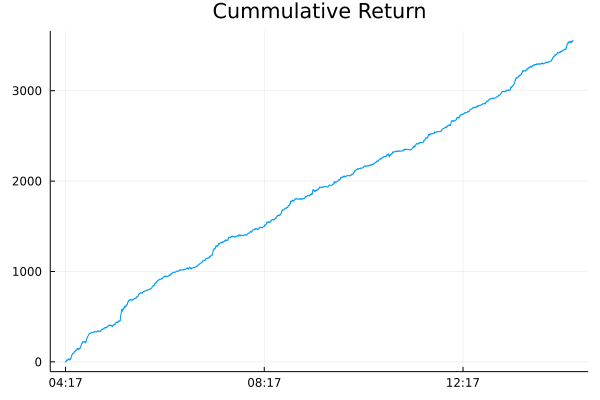Up and to the right as we would hope. So following this strategy would make you money. Theoretically. But is it a good strategy? To measure this we can calculate the Sharpe ratio, which is measuring the overall profile of the returns compared to the volatility of the returns.

moneyReturns = sign.(testData.CloseClosePred) .* testData.CloseCloseReturn
mean(moneyReturns) ./ std(moneyReturns)

0.11599938576235787


A Sharpe ratio of 0.12 if we are generous and round up. Anyone with some experience in these strategies is probably having a good chuckle right now, this value is terrible. At the very minimum, you would like a value of 1, i.e. that your average return is greater than the variance in returns, otherwise you are just looking at noise.

How many times did we correctly guess the direction of the market though? This is the hit ratio of the strategy.

mean(abs.((sign.(testData.CloseClosePred) .* sign.(testData.CloseCloseReturn))))

0.530163738236414


So 53% of the time I was correct. 3% better than a coin toss, which is good and shows there is a little bit of information in the OFI values when predicting.

## Does a Threshold Help?

Should we be more selective when we trade? What if we set a threshold and only trade when our prediction is greater than that value. Plus the same in the other direction. We can iterate through lots of potential thresholds and see where the Sharpe ratios end up.

p = plot(ylabel = "Cummulative Returns", legend=:topleft, fmt=:png)
sharpes = []
hitratio = []

for thresh in 0.01:0.01:0.99
trades = sign.(testData.CloseClosePred) .* (abs.(testData.CloseClosePred) .> thresh)

newMoneyReturns = trades .* testData.CloseCloseReturn

sharpe = round(mean(newMoneyReturns) ./ std(newMoneyReturns), digits=2)
hr = mean(abs.((sign.(trades) .* sign.(testData.CloseCloseReturn))))

if mod(thresh, 0.2) == 0
plot!(p, testData.timestampfloor, cumsum(newMoneyReturns), label="\$(thresh)", xticks = (xticks, xtickslabels))
end
push!(sharpes, sharpe)
push!(hitratio, hr)
end
p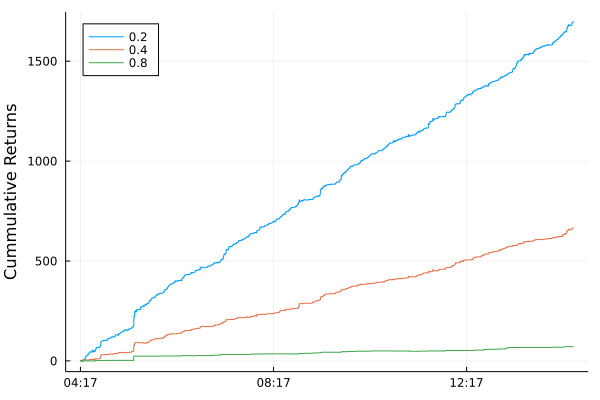The equity curves look worse with each higher threshold.

plot(0.01:0.01:0.99, sharpes, label=:none, title = "Sharpe vs Threshold", xlabel = "Threshold", ylabel = "Sharpe Ratio", fmt=:png)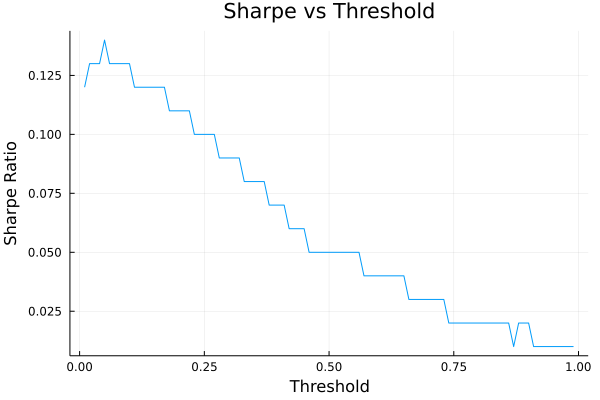A brief increase in Sharpe ratio if we set a small threshold, but overall, steadily decreasing Sharpe ratios once we start trading less. For such a simple and linear model this isn’t surprising, but once you start chucking more variables and different modeling approaches into the mix it can shed some light on what happens around the different values.

## Why you shouldn’t trade this model

So at the first glance, the OFI signal looks like a profitable strategy. Now I will highlight why it isn’t in practice.

• Trading costs will eat you alive

I’ve not taken into account any slippage, probability of fill, or anything that a real-world trading model would need to be practical. As our analysis around the Sharpe ratio has shown, it wants to trade as much as possible, which means transaction costs will just destroy the return profile. With every trade, you will pay the full bid-ask spread in a round trip to open and then close the trade.

• The Sharpe ratio is terrible

With a Sharpe ratio < 1 shows that there is not much actual information in the trading pattern, it is getting lucky vs the actual volatility in the market. Now, Sharpe ratios can get funky when we are looking at such high-frequency data, hence why this bullet point is second to the trading costs.

• It has been trained on a tiny amount of data.

Needless to say, given that we are looking at seconds this dataset could be much bigger and would give us greater confidence in the actual results once expanded to a wider time frame of multiple days.

• I’ve probably missed something that blows this out of the water

Like everything I do, there is a strong possibility I’ve gone wrong somewhere, forgotten a minus, ordered a time-series wrong, and various other errors.

## How this model might be useful

• An overlay for a market-making algorithm

Making markets is about posting quotes where they will get filled and collecting the bid-ask spread. Therefore, because our model appears to be able to predict the direction fairly ok, you could use it to place a quote where the market will be in one second, rather than where it is now. This helps put your quote at the top of the queue if the market does move in that direction. Secondly, if you are traded with and need to hedge the position, you have an idea of how long to wait to hedge. If the market is moving in your favour, then you can wait an extra second to hedge and benefit from the move. Likewise, if this model is predicting a move against your inventory position, then you know to start aggressively quoting to minimise that move against.

• An execution algorithm

If you are potentially trading a large amount of bitcoin, then you want to split your order up into lots of little orders. Using this model you then know how aggressive or passive you should trade based on where the market is predicted to move second by second. If the order flow imbalance is trending positive, the market is going to go up, so you want to increase your buying as not to miss out on the move and again, if the market is predicted to move down, you’ll want to slow down your buying so that you fully benefit from the lull.

## Conclusion

Overall hopefully you now know more about order flow imbalance and how it can somewhat explain returns. It also has some predictive power and we use that to try and build a trading strategy around the signal.

We find that the Sharpe ratio of said strategy is poor and that overall, using it as a trading signal on its own will not have you retiring to the Bahamas.

This post has been another look at high-frequency finance and the trials and tribulations around this type of data.